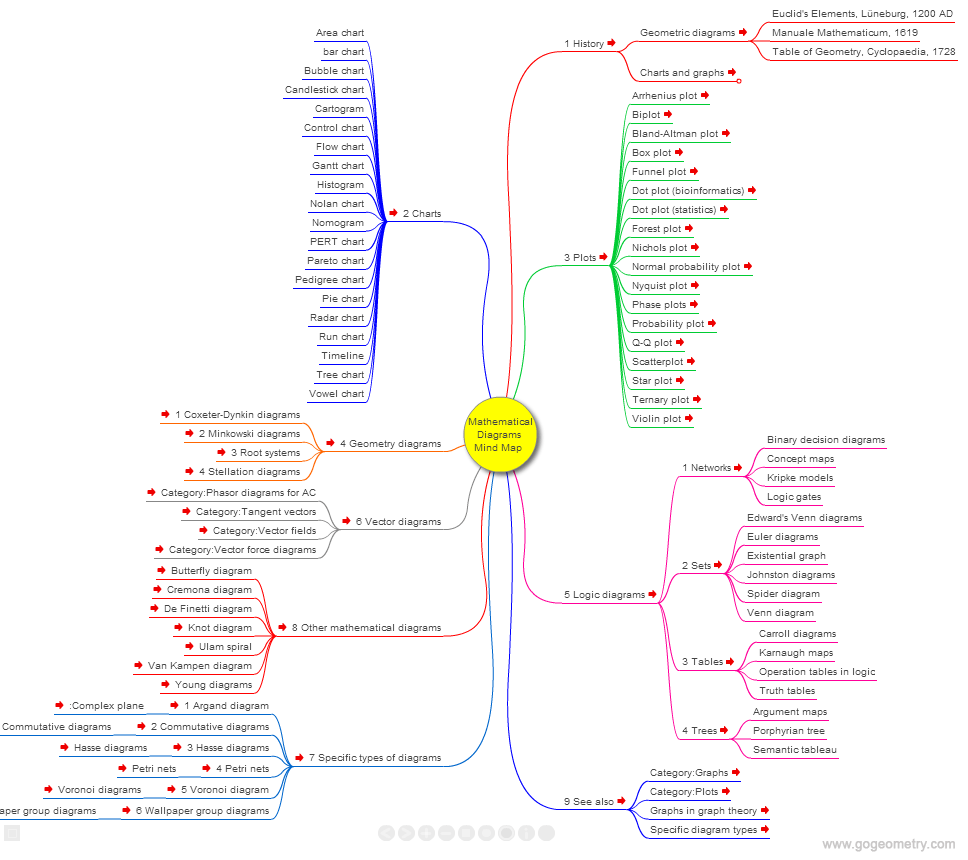# Mathematical Diagrams, Interactive Mind MapMind Map Description

Interactive Mind Map that represents connections between various topics and concepts related to Mathematical Diagram. Source: Wikipedia: Mathematical Diagram.

Mathematic diagrams
Mathematic diagrams are diagrams in the field of mathematics, and diagrams using mathematics such as charts and graphs, that are mainly designed to convey mathematical relationships.

Graphic organizers
Graphic organizers are visual representations of knowledge, concepts or ideas.Data Visualization

Engineering Drawings Mind Map
Visualization of Technical Information, Mind Map
Data Visualization, Mind Map
Technical Drawings Mind Map
Mathematical Diagrams Mind Map
Information Mapping, Mind Map

Home | Search | Mind Map Index | Math Mind Map Index | Visualization | Education | Post a comment | Email | by Antonio Gutierrez